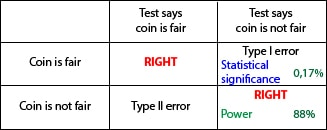# The false coin

Today we’re going to continue playing with coins. In fact, we’re going to play with two coins, one of them a fair coin and the other one faker than Judas Iscariot, loaded to give more heads than tails when flipped. I recommend you to sit back and relax before starting.

It turns out we have a loaded coin. By definition, the probability of getting heads when tossing a fair coin is 0.5 (50%). However, our fake coin lands on heads 70% of the time (probability 0.7), which comes in handy because we can use it whenever we want to negotiate any unpleasant task. We only have to offer our coin, choose tails and trust to be lucky enough to be benefited by our unfair coin.

Let’s suppose now we have been so careless as to put the fake coin with the others. How can we know what is the false one?. And this is when we think about our game. Let’s imagine what would happen if we flipped a coin 100 times in a row. If the coin is fair we expect to get heads 50 times, whereas if the coin was our false one, we’d expect 70 heads. So we can choose a coin at random, toss it 100 times and, counting the number of heads, decide if it’s fair or not. We can arbitrarily choose a value between 50 and 70, let’s say 65, and state: if we get 65 heads or more our coin will be the loaded one, but if we get less than 65, we’ll say it is a fair coin.

But anyone immediately realizes that this method is not foolproof. On the one hand, we can get 67 heads with a fair coin and conclude it’s not, when it is indeed fair. But it can also happen that, just by chance, we get 60 heads with the loaded coin and conclude it is fair. Can we solve this problem and avoid getting at the wrong conclusion?. Well, the truth is that we can’t, but what we can do is to measure the likelihood we have of making a mistake.

If we use a binomial probability calculator (the bravest of you can do the calculations by hand) we’ll come up with a probability of getting 65 heads or more with a fair coin of 0.17%, while the probability of getting them with the loaded coin is 88.4%. So we can find ourselves four possibilities that I represent in the accompanying table.In this case, our null hypothesis says that the coin is fair, while the alternative hypothesis says that the coin is spoofed in favor of heads.

Let’s start with the case the test concludes that the coin is fair (we get less than 65 heads). The first possibility is that the coin is actually fair. Well, we’ll be right. We have no more to say about that situation.

The second possibility is that, despite the conclusion of our test, the coin is faker than the kiss of a mother-in-law. Well, this time we’ll have made a mistake that someone with little imagination named as type II error. We have accepted the null hypothesis that the coin is fair when it’s actually unfair.

We’re going to suppose now that our test concludes that the coin is loaded. If the coin is actually fair, we will err again, but this time we will have committed a type I error. In this case, we reject the null hypothesis that the coin is fair when it is actually fair.

Finally, if we conclude that it is not fair and it is actually loaded, we will be right again.

We can see in the table that the probability of making a type I error is, in this example, 0.17%. This is the statistical significance level of our test, which is just the probability of rejecting our null hypothesis that the coin is fair (concluding it is false) when it is in fact fair. On the other hand, the probability of being right when the coin is false is 88%. This probability is called the power of the test, and it is just the probability of being right when the test concludes the coin is loaded (put it in other words, reject the null hypothesis and be right).

If you think a little about it you will see that the type II error is the complementary of power. When the coin is not fair, the probability of accepting it is fair (type II error) plus the probability of being right and conclude it is false must add up to 100%. Thus, type II error equals 1 minus power.

This statistical significance we have seen is the same as the famous p value. Statistical significance is just the probability of committing a type I error. By convention, it’s generally accepted as tolerable when it is less than 0.05 (5%) since, in general, it is preferable not to accept a false hypothesis. This is why scientific studies look for low values of significance and high values for power, although both of them are related, so that increasing significance decreases power and vice versa.

And this is the end for now. Those of you that have got this far through this rigmarole without getting missing at all, my sincere congratulations, because the truth is that this post seems a play on words. And we could have said something about significance and the calculation of confidence intervals, samples sizes, etc. But that’s another story…

This site uses Akismet to reduce spam. Learn how your comment data is processed.